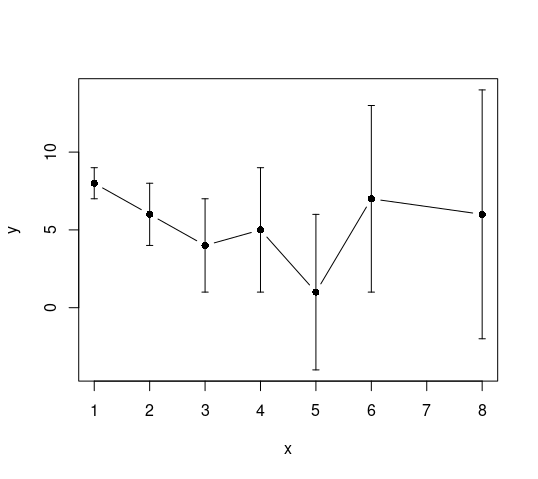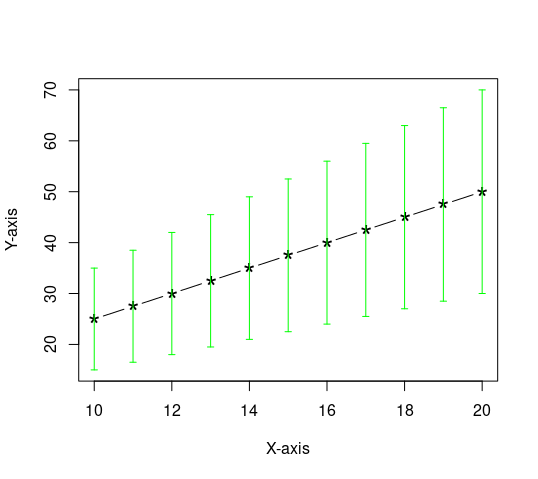# Line Plot in R with Error Bars

• Last Updated : 24 Dec, 2021

A line plot is a graphical representation of a series of data. Line plots are widely used in data visualization. In we use ggplot2( ) and plot( ) function to create line plot. Error bars act as a visual enhancement that help us to see variability of the plotted data. Error Bars are used to show standard error, standard deviation i.e. with the help of integrated error bars in our plot we can know the estimated error.

In this article we are going to create a line plot with Error Bars and without Error Bars. We can use plot() function to create scatter plot and line plot also.

Syntax:

errbar(x, y, yplus, yminus, cap=0.015, main = NULL, sub=NULL, xlab=as.character(substitute(x)), ylab=if(is.factor(x) || is.character(x)) ” “else as.character(substitute(y)), add=FALSE, lty=1, type=’p’, ylim=NULL,pch, lwd=1, errbar.col, )

Where,

### Approach:

• Firstly open up your rstudio
• Type the following command and hit enter to install the dependency that will help us to draw error bars in line plots.
`install.packages("Hmisc")`
• Load library by typing :
`library(Hmisc)`
• Create two data variables one for the x-axis and another for the y-axis
• Create a vector to store error values (randomly)
• Show error bars using errbar()

Example 1:

## R

 `# Creating random data` `x = ``c``(1,2,3,4,5,6,8)` `y = ``c``(8,6,4,5,1,7,6) `   `# generating error_values` `error_values = ``c``(x)`   `# plotting` `errbar``(x,y,y+error_values,y-error_values,type=``'b'``)`

Output :Fig. 1. Simple line plot with error bars.

Example 2:

## R

 `# Creating random data` `x = 10:20` `y = x*2.5`   `# generating error_values` `# you can use any custom` `# function to calculate error` `error_values = ``sqrt``(y)`   `# plotting` `errbar``(x,y,y+error_values,y-error_values,type=``"b"``,` `       ``col=``'black'``,cex=2,errbar.col=``'green'``,` `       ``xlab=``"X-axis"``,ylab=``"Y-axis"``,` `       ``pch=``"*"``)`

Output:Fig. 2. Custom line plot with error bars.

My Personal Notes arrow_drop_up
Recommended Articles
Page :